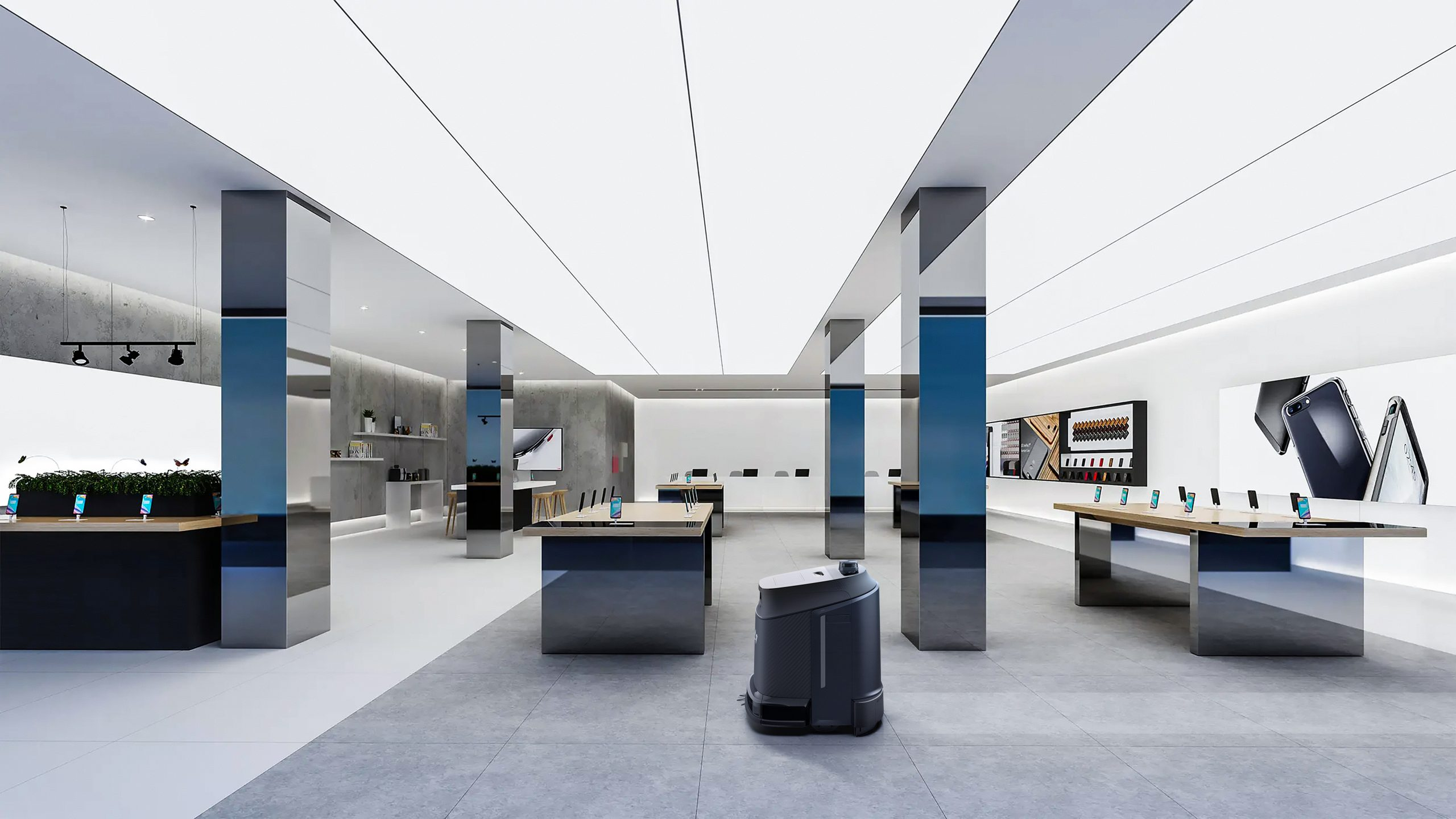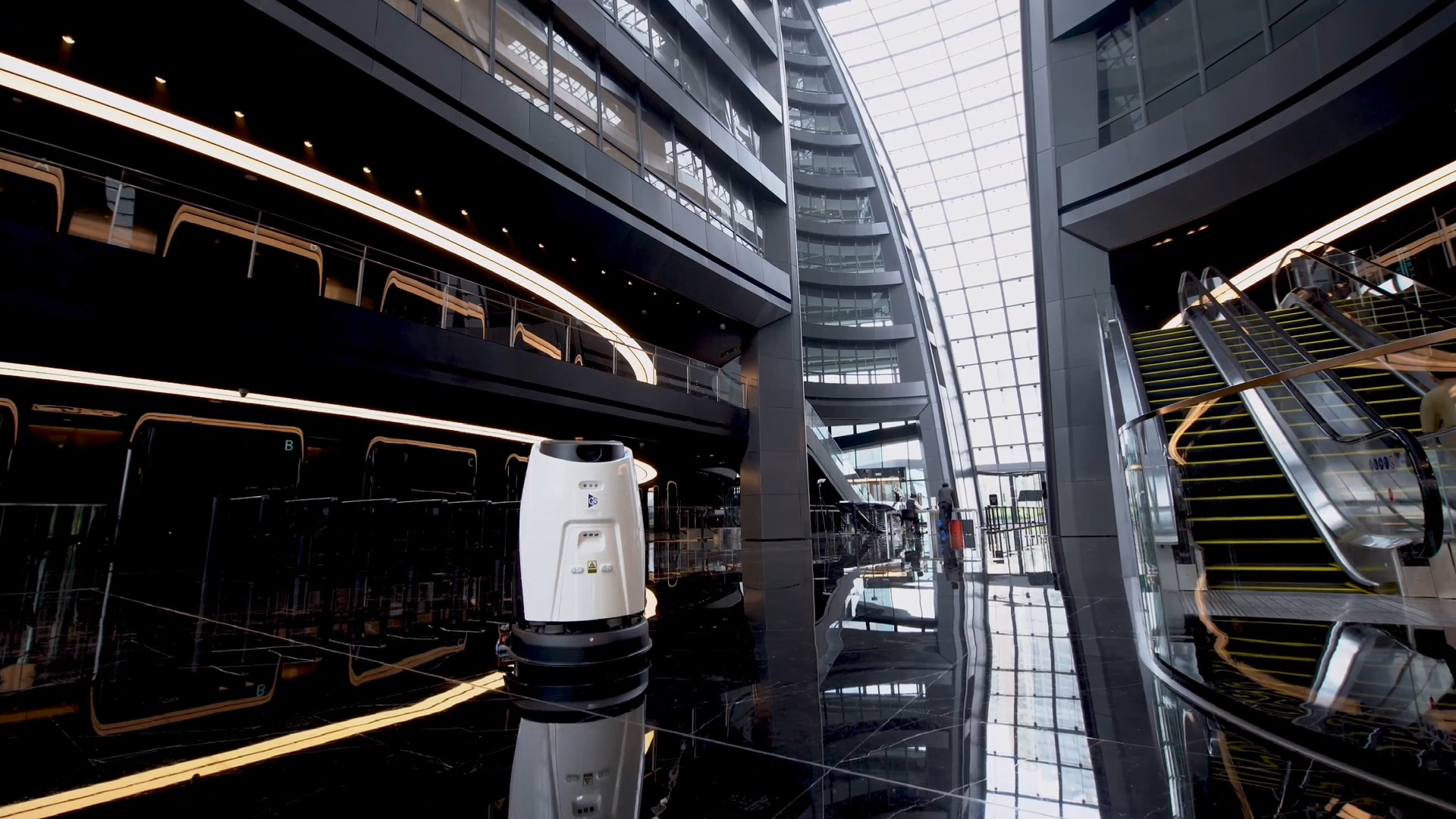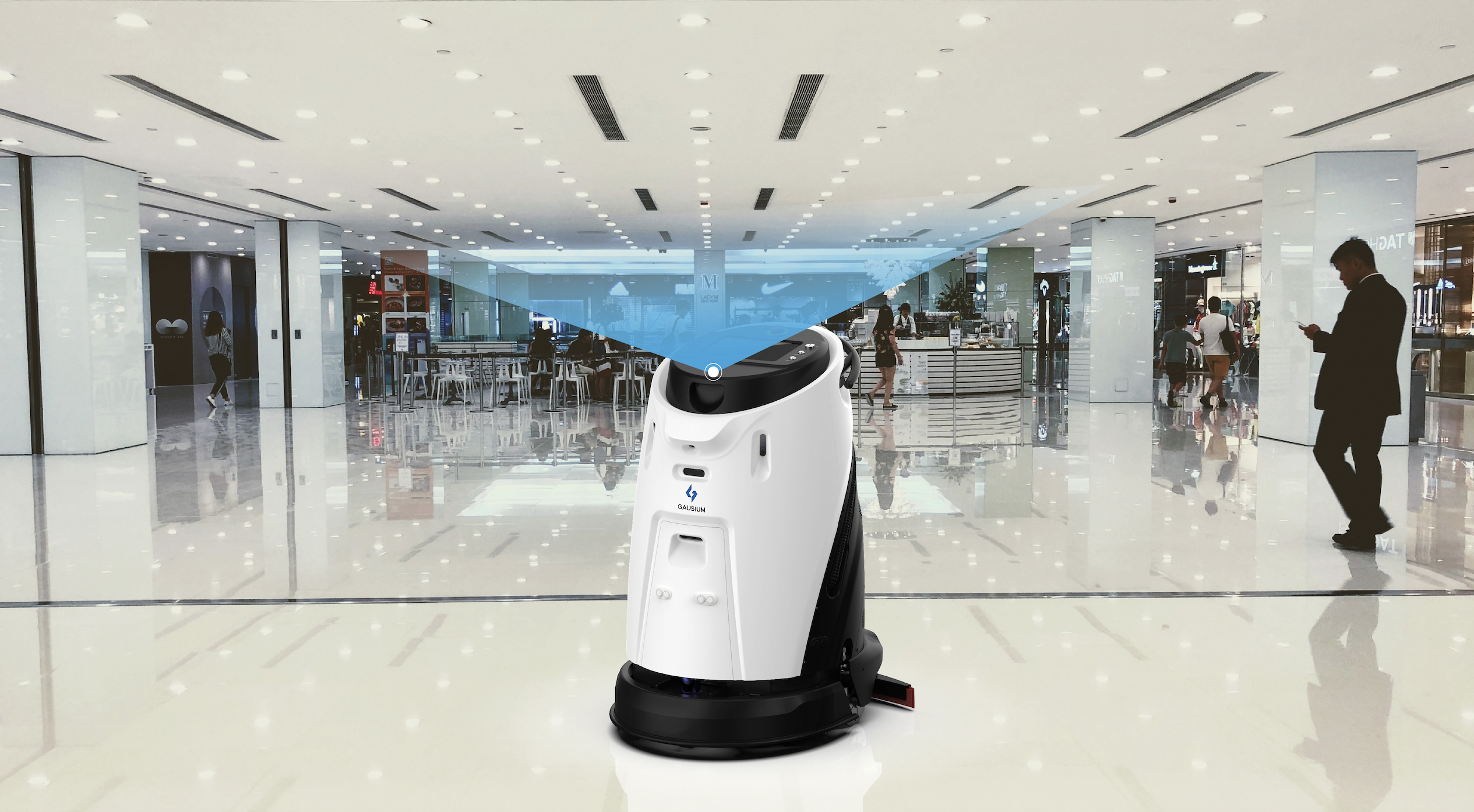WordPress資料庫錯誤： [“where子句”中“weglot”一欄未知]
``` SELECT `meta_key` AS `key`, `meta_value` AS `value` FROM `ga_postmeta` WHERE `post_id` = weglot-4449-en AND ( `meta_key` = "_en_item_image" OR `meta_key` = "_en_item_image_hover" ) ```

WordPress資料庫錯誤： [“where子句”中“weglot”一欄未知]
``` SELECT `meta_key` AS `key`, `meta_value` AS `value` FROM `ga_postmeta` WHERE `post_id` = weglot-4449-es AND ( `meta_key` = "_en_item_image" OR `meta_key` = "_en_item_image_hover" ) ```

WordPress資料庫錯誤： [“where子句”中“weglot”一欄未知]
``` SELECT `meta_key` AS `key`, `meta_value` AS `value` FROM `ga_postmeta` WHERE `post_id` = weglot-4449-fr AND ( `meta_key` = "_en_item_image" OR `meta_key` = "_en_item_image_hover" ) ```

WordPress資料庫錯誤： [“where子句”中“weglot”一欄未知]
``` SELECT `meta_key` AS `key`, `meta_value` AS `value` FROM `ga_postmeta` WHERE `post_id` = weglot-4449-ko AND ( `meta_key` = "_en_item_image" OR `meta_key` = "_en_item_image_hover" ) ```

WordPress資料庫錯誤： [“where子句”中“weglot”一欄未知]
``` SELECT `meta_key` AS `key`, `meta_value` AS `value` FROM `ga_postmeta` WHERE `post_id` = weglot-4449-ja AND ( `meta_key` = "_en_item_image" OR `meta_key` = "_en_item_image_hover" ) ```

WordPress資料庫錯誤： [“where子句”中“weglot”一欄未知]
``` SELECT `meta_key` AS `key`, `meta_value` AS `value` FROM `ga_postmeta` WHERE `post_id` = weglot-4449-zh AND ( `meta_key` = "_en_item_image" OR `meta_key` = "_en_item_image_hover" ) ```

WordPress資料庫錯誤： [“where子句”中“weglot”一欄未知]
``` SELECT `meta_key` AS `key`, `meta_value` AS `value` FROM `ga_postmeta` WHERE `post_id` = weglot-4449-it AND ( `meta_key` = "_en_item_image" OR `meta_key` = "_en_item_image_hover" ) ```

WordPress資料庫錯誤： [“where子句”中“weglot”一欄未知]
``` SELECT `meta_key` AS `key`, `meta_value` AS `value` FROM `ga_postmeta` WHERE `post_id` = weglot-4449-de AND ( `meta_key` = "_en_item_image" OR `meta_key` = "_en_item_image_hover" ) ```

WordPress資料庫錯誤： [“where子句”中“weglot”一欄未知]
``` SELECT `meta_key` AS `key`, `meta_value` AS `value` FROM `ga_postmeta` WHERE `post_id` = weglot-4449-nl AND ( `meta_key` = "_en_item_image" OR `meta_key` = "_en_item_image_hover" ) ```

• 產品亮點

# Gausium 3 年 2022 篇熱門文章

12月26， 2022

1. 適合中小型商業地面清潔的最佳機器人解決方案1. 更加智能的全自動清潔機器人1. 機器人永不迷路——Gausium 無碼部署的天花板視覺定位系統